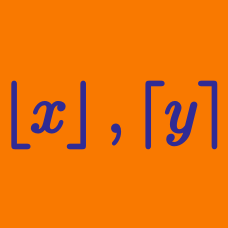Algebra

# Fractional Part Function

What is $\left\{\sqrt{71}\right\}\times\left(\sqrt{71}+8\right)?$

Note: $\{x\}$ denotes the fractional part function.

What is $\{21.11\}?$

Note: $\{x\}$ denotes the fractional part function.

If $0 how many solutions are there to $\{x\}=0.9?$

Note: $\{x\}$ denotes the fractional part function.

What is $\{15.15\}+\{-15.15\}?$

Note: $\{x\}$ denotes the fractional part function.

What is $\left\{\frac{85}{6}\right\}?$

Note: $\{x\}$ denotes the fractional part function.

×